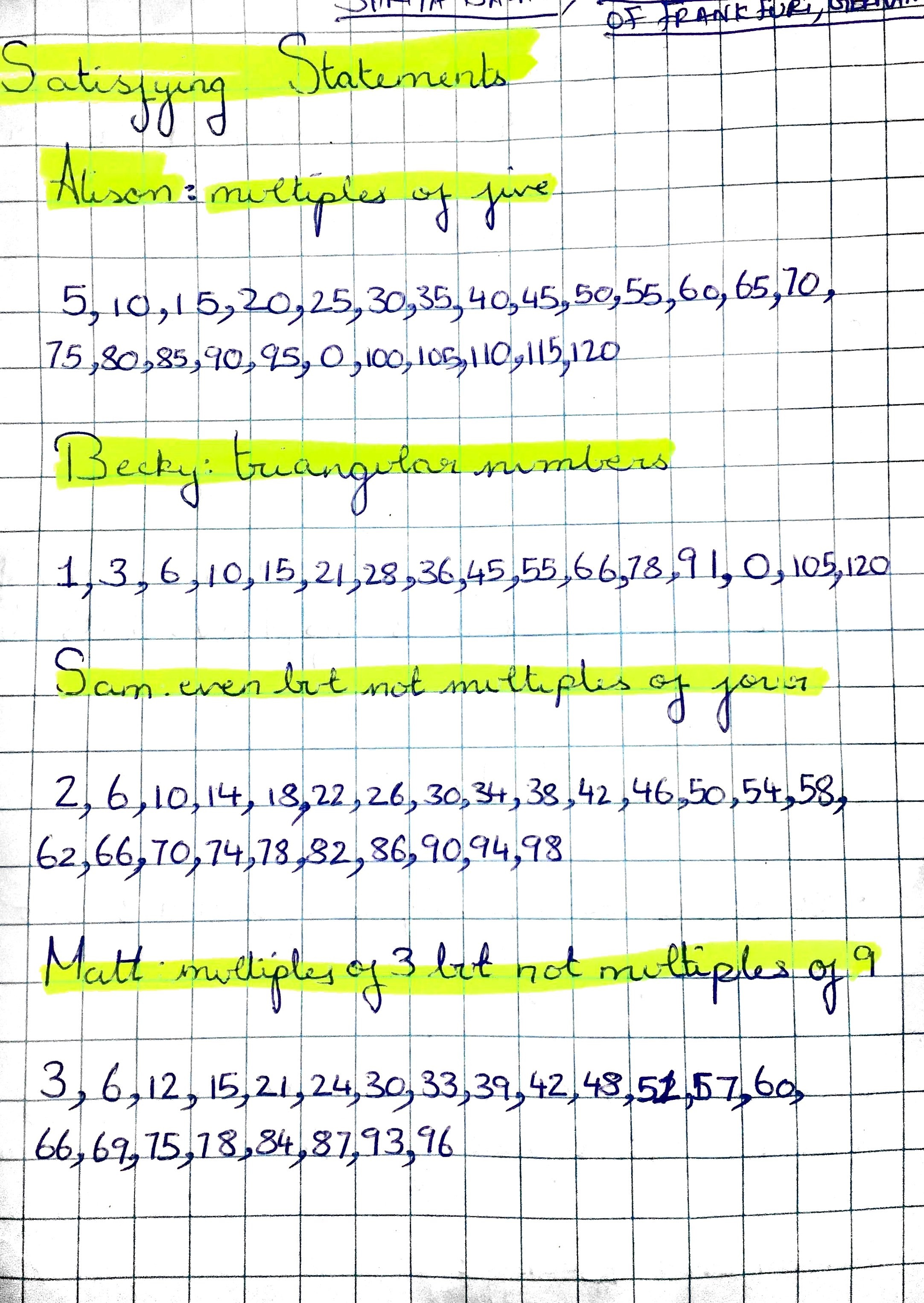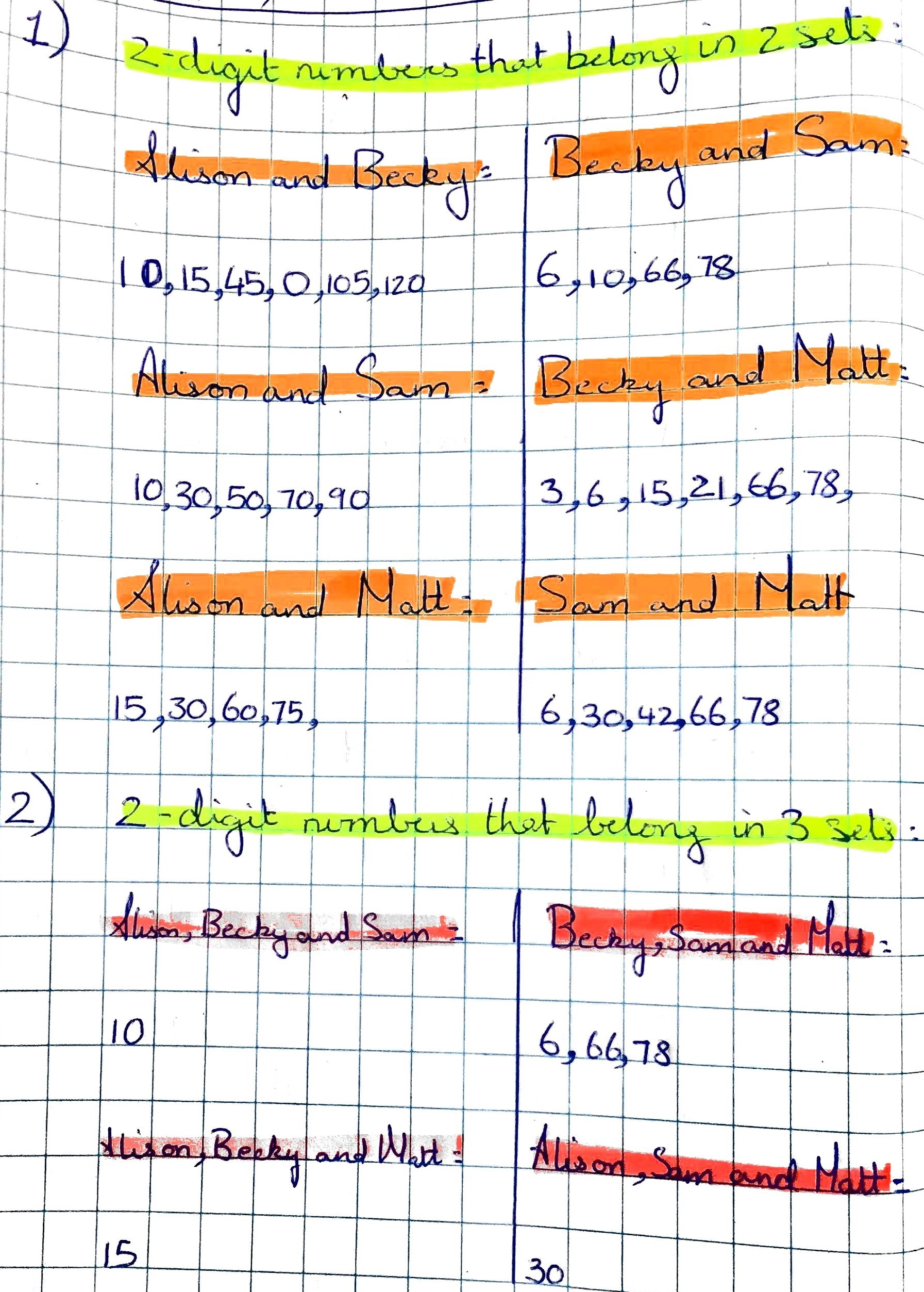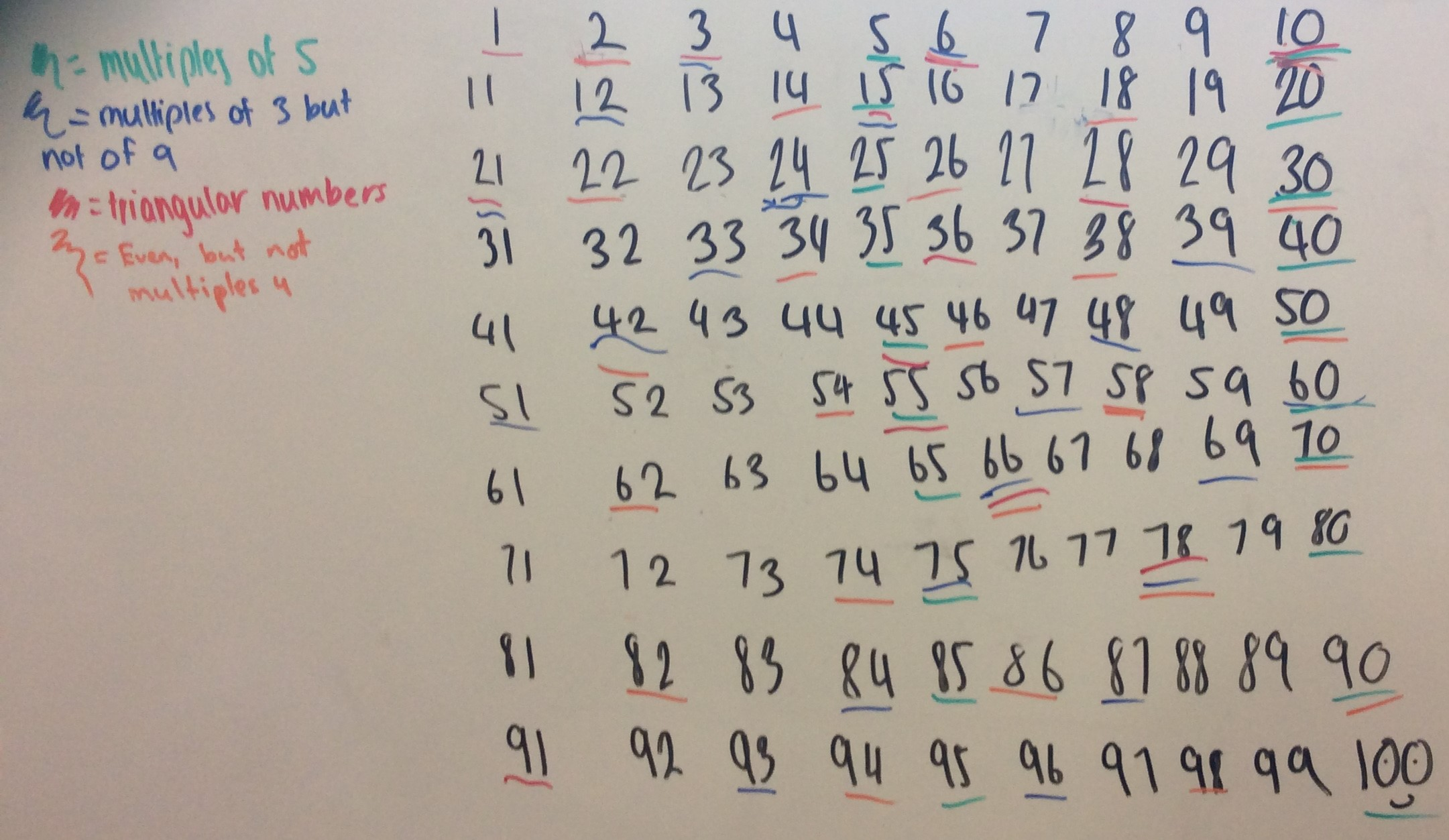#### You may also likeMake a set of numbers that use all the digits from 1 to 9, once and once only. Add them up. The result is divisible by 9. Add each of the digits in the new number. What is their sum? Now try some other possibilities for yourself!### Have You Got It?

Can you explain the strategy for winning this game with any target?### Counting Factors

Is there an efficient way to work out how many factors a large number has?

# Satisfying Statements

##### Age 11 to 14Challenge Level

Well done and thank you to everyone who submitted a solution. There were too many good solutions to mention everyone, but this is a selection of the good work we received.

Anton from Brooklands Primary School in England found the smallest numbers that satisfy some pairs and groups of statements:
A and B smallest is 10
A and S smallest is 10
A and M smallest is 15
B and S smallest is 6
S and M smallest is 6
A and B and S smallest is 10
A and S and M smallest is 30

Lexi found larger numbers that belong in more than one set:
66, even but but not a multiple of 4 and triangular number.
21, multiple of 3 and not a multiple of 9 and triangular number.
595, multiple of 5, multiple of 3 but not 9 and triangular number.
465, multiple of 5, multiple 3 but not 9, and a triangular number.
435, multiple of 5, multiple of 3 but not 9, and triangular number.

Peter from Highcrest Middle School in the USA found that 5050 satisfied all of the statements.

Shriya from International School Frankfurt in Germany listed numbers which satisfied each statement to find numbers that appeared more than once.Ailish, Courtney and Nathan from Sirius Academy North, Hull, UK found the smallest number that belongs in all 4 sets. They eliminated numbers that failed to satisfy one of the statements.
First we wrote down the first 20 triangular numbers.
Then we eliminated ones that aren't multiples of 5.
Then we eliminated the odd ones, then the ones that were multiples of 4.
This took us to just having 190 and 210 left.
Finally we eliminated 190 because it was not a multiple of 3. 210 is a multiple of 3 but not a multiple of 9 so that's the smallest number in all 4 sets!

Vanshika from Rishi Valley School in India used a similar method, but by writing new lists rather than by eliminating numbers:
So what I do is I lay my numbers on the sheet .
Q1 has to be a multiple of 5
Q2 has to be a triangle no.
Q3 has to be even but not divisible by 4
Q4 has to be a multiple of 3 but not 9

So for the first one
5, 10, 15, 20, 25, 30, 35, 40, 45, 50, 55, 60, 65, 70, 75, 80, 85, 90, 95, 100, ...
Second, but now it has to be a triangle number too
10, 45, 55, 105, 120, 190, 210, 300
Third, now it has to be even but not a multiple of 4
10, 190, 210
Fourth, now it has to be a multiple of 3 but not 9
210

Sophie from Stephen Perse Foundation in the UK sent in a very clear and full solution up to this point. Click here  to see Spohie's work.

Maysa, Rizqin and Soyeon from Greenacre Public School in Australia sent in an image:
The way we did it was to write a 1-100 chart and colourcoded it according
to the statements it satisfied, and that was our answerChloe from Harrow International School Hong Kong used properties of numbers to find a more efficient method:
To find the smallest number that fits into all four sets, one way to do it would be to extend all the lists to find a number which occurs in all four lists. However, that would take a long time.

As this number has to be a multiple of five and even, we know the last digit must be a zero. It also needs to be divisible by three and not nine.

We can narrow this down using the above list for the numbers divisible by 3 but not 9:

3, 6, 12, 15, 21, 24, 30, 33, 39, 42, 48, 51, 57, 60, 66, 69, 75, 78”¦ etc.

The first or first two digits of the smallest number that belongs to all four of the groups must be from the above list.
The smallest triangular numbers that end in 0 are: 10, 120, 190, 210, 300

Of these, the only ones divisible by three and not nine, and are even but not multiples of 4 are:
210 and 300

The smallest number that belongs to all four groups is therefore 210.

Vid from Primary School Loka ÄŒrnomelj and Matthew from Harrow International School Hong Kong both used a similar method to Chloe's, but with more algebra. This is a mixture of their work:
Let's write a formula for a general number in each set.
Alison: $5n$
Becky: $\frac{n(n+1)}2$
Sam: $4n-2$
Matt: As there is no distinct formula, we could simply use 3n, or we could use two: $9n_1-6$ or $9n_2-3$. These can be written as $3(3n_1-2)$ and $3(3n_2-1)$.

We see, that the number we are looking for is certainly a multiple of $5\times2\times 3 = 30$. However, it must not be divisible by $9$ or $4$, so it must not be $30$ mutliplied by a multiple of $2$ or $3$.

So, $\frac{n(n+1)}2$ has to be a multiple of $30$.

Rules for numbers in more than one set
Alison and Sam

Aryaman from Bangkok Patana School in Thailand found two ways of describing these numbers:
List the numbers that satisfy both Alison's and Sam's statements:
{10,30,50,70,90,110,130,150,...}

One way to describe it would be: start at 10 and go up by 20.
Another would be to list odd numbers and put a '0' to at the end of each.
For example:
1,3,5,7,9,... but we put a '0' at the end: 10,30,50,70,90,110,...

Rainbow explained why this works, using Aryaman's first description:
The pattern for Alison & Sam's numbers are that they increase by 20 each time. The reason you have to begin this sequence with the number 10 is because no number less than 10 is both a multiple of 5 and also an even number which is not a multiple of 4.They can't increase by 5 or 15 because the number would therefore end in a 5, making it an odd number. Neither can they increase by 10 each time because it specifically says that it cannot be a multiple of 4. Because 4 is a factor of twenty, it is obviously also a factor of all multiples of 20. If you add 10 to 10, that would result in the number 20 (so if you add 10 to the first number in the sequence, you get a multiple of 4) and if you add 10 to 30 (the next number in the sequence) it would make 40, a multiple of 4.

Garrett from Harrow International School Hong Kong explained why this works, using Aryaman's second description:
Since the last digit has to be even and a digit that is a multiple of 5, the last digit will have to be 0, but since the last two digits cannot be divisible by 4, in the last 2 digits, the first digit has to be an odd number and the last digit has to be 0.

Year 8 at Badminton School in the UK found an expression for a general number which fits in both sets:
$20n-10$

Damian from Harrow International School in Hong Kong wrote this as:
Multiple of 5 and being even would be a multiple of 10, and not being a multiple of 4 would mean not being a multiple of 20.
So they are multiples of 10 but not multiples of 20.

Alison and Matt
Hannah, Matthew, Fred, Lucas and Liam from Balmoral School in New Zealand said:

When we looked at the relationship between A and M, they contain every first and second multiple of 15, but not the third. The pattern restarts so 15, 30, 60,75, 105 etc. This is because the third, sixth, ninth etc multiple of 15 is divisible by 9.

Damian wrote this as:
Multiple of 5 and multiple of three would be multiples of 15, and not being a multiple of 9 would mean not being a multiple of 45.
So they are multiples of 15 but not multiples of 45.

Alison and Becky
Shriya said:

It should be a multiple of 5
It should be a triangular number
Therefore the numbers are 10, 15, 45, ...

Someone known as XYZ from Green Oasis School in China said:
The numbers that satisfy Becky's statement follow this description $\frac{n\times(n+1)}2$
To satisfy Alison and Becky's statement, either $n$ or $n+1$ should be a multiple of five.
We get the following set of numbers: 10 15 45 55

Gina from Wimbledon High School in the UK said:
10, 15, 45, 50 etc.
Fit Alison's and Becky's statements. Every 4th and 5th triangular numbers fit both of their descriptions.

Notice that if $n$ is a 4th or 5th number, then $n+1$ or $n$ is respectively a multiple of 5. So this is how XYZ's observation explains Gina's observation.

Sam and Matt
Ashlynn from ISF Academy in Hong Kong said:

Numbers that belong to Sam and Matt's set: 6, 30, 42”¦
Therefore, the numbers are multiples of 6, but not multiples of 9 and 4.

Damian described this as:
Even and multiple of three would be multiples of 6, and not being multiples of four and nine would mean not being a multiple of 12 or 18 respectively.
So these numbers are multiples of 6 but not multiples of 9 or 18.

Becky and Sam
Kevin from Harrow International School in Hong Kong found some numbers in both of these sets:

Triangular numbers that are even, but not multiples of 4
(eg. 66, 78, 190)

Georgie from Stephen Perse Foundation in the UK noticed that:
Every seven triangular numbers, there is a pair, e.g. 6, 10, ..., 66, 78, ... Between these pairs, there is 4, then 12, then 20, etc. this goes up in eights (because you go up by 7 triangular numbers to reach the next pair, and the difference between each pair of consecutive triangular numbers is always 1 more than the difference between the pair before it)

Damian said:
By looking at the sequence of triangular numbers, you would find that the first four of these would be the 3rd, 4th, 11th and 12th triangular number. Continuing the sequence, you would get the 19th, 20th, 27th and 28th triangular number. Therefore, by starting at three, and adding 1 whenever your current number is odd and adding 7 when it's even, you would get all the terms that satisfy Becky and Sam's statements. The pattern would go 6, 10, 66, 78, 190 etc etc etc, with these numbers being the 3rd, 4th, 11th, 12th and 19th term respectively.

Becky and Matt
Georgie noticed that:

Every one, then four, then eight, etc triangular numbers there is a pair, e.g. 3, 6, ..., 15, 21, ..., 66, 78, ..., 210, 231.